# Maya math node summary# Maya math nodes

A small list of useful Maya nodes, I only list some of the most useful math nodes here. You can find the full list of Maya nodes in the technical documentation.

## Expressions

If you don't find the native Maya node you are looking for, Maya animation expressions might do the job. Expression nodes are notoriously slow but allows for quick prototyping, also keep in mind that it is named based and any object renaming will potentially break your expressions.

A list of available functions inside an expression:
- math functions: trunc(), ceil(), floor() clamp(min, max, value), sin(), cos(), tan(), min(), max(), sqrt(), exp(), log(), sign() (and other trig functions ...)
- Random functions: gauss(), noise(), dnoise(), rand(), sphrand(), seed()
- Vector functions: angle(), cross(), dot(), mag(), rot(), unit()
- Array functions: clear(), size(), sort()
- Curve functions: linstep(), smoothstep(), hermite()(vector), hermite()(scalar)

## Bifrost

Bifrost is a powerful node system usually known for its ability to simulate fluids, smokes and such, but that can be used to work on geometry as well, and provides all the basic and more advanced math nodes and help you with rigging.

## Native nodes

plusMinusAverage math node specification. Originally designed with shaders in mind those operations work on vectors, i.e. addition, division etc is usually applied on a per component basis (out.x = in1.x + in2.x), (out.y = in1.y + in2.y) etc.

 Note: when disconnecting make sure to reset the value of the attribute, otherwise it will keep adding, subtracting etc. whatever was the value at disconnection. SUM:     Output1D = $$\sum_i$$ Input1D[ i ]     Output2Dx = $$\sum_i$$ Input2D[ i ]x     Output2Dy = $$\sum_i$$ Input2D[ i ]y     Output3Dx = $$\sum_i$$ Input3D[ i ]x     Output3Dy = $$\sum_i$$ Input3D[ i ]y     Output3Dz = $$\sum_i$$ Input3D[ i ]z SUBSTRACT:     Output1D = Input1D[ 0 ] - $$\sum_{i+1}$$ Input1D[ i ]     Output2Dx = Input2Dx[ 0 ] - $$\sum_{i+1}$$ Input2Dx[ i ]     Output2Dy = Input2Dy[ 0 ] - $$\sum_{i+1}$$ Input2Dy[ i ]     Output3Dx = Input2Dx[ 0 ] - $$\sum_{i+1}$$ Input2Dx[ i ]     Output3Dy = Input2Dy[ 0 ] - $$\sum_{i+1}$$ Input2Dy[ i ]     Output3Dz = Input2Dz[ 0 ] - $$\sum_{i+1}$$ Input2Dz[ i ] AVERAGE: Note: when disconnecting make sure to delete the array element otherwise it will average based on the current size.     N = getAttr -size plusMinusAverage.Input1D     Output1D = ( $$\sum_i$$ Input1D[ i ] ) / N     N = getAttr -size plusMinusAverage.Input2D     Output2Dx = ( $$\sum_i$$ Input2D[ i ]x ) / N     Output2Dy = ( $$\sum_i$$ Input2D[ i ]y ) / N     N = getAttr -size plusMinusAverage.Input3D     Output3Dx = ( $$\sum_i$$ Input3D[ i ]x ) / N     Output3Dy = ( $$\sum_i$$ Input3D[ i ]y ) / N     Output3Dz = ( $$\sum_i$$ Input3D[ i ]z ) / N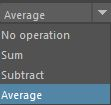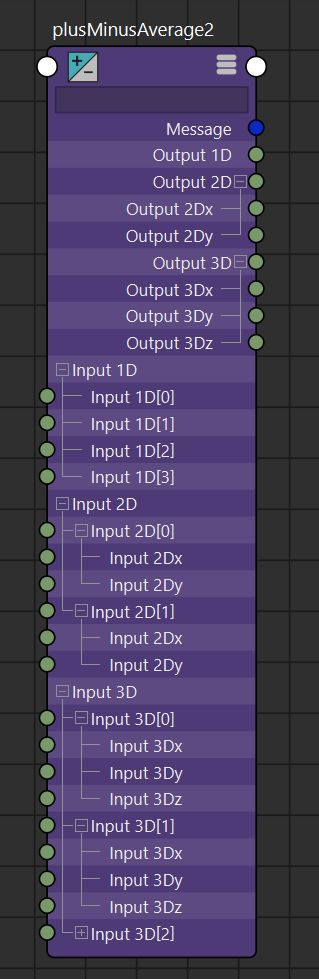divideMultiply math node specification.

 NO OPERATION:     Output.x = Input1.x     Output.y = Input1.y     Output.z = Input1.z     Input2.xyz is ignored. MULTIPLY:     Output.x = Input1.x * Input2.x     Output.y = Input1.y * Input2.y     Output.z = Input1.z * Input2.x DIVIDE:     Output.x = Input1.x / Input2.x     Output.y = Input1.y / Input2.y     Output.z = Input1.z / Input2.x POWER:     Output.x = $$(Input1.x) ^ { Input2.x }$$     Output.y = $$(Input1.y) ^ { Input2.y }$$     Output.z = $$(Input1.z) ^ { Input2.x }$$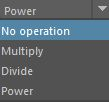condition math node specification. Compare 'firstTerm' and 'secondTerm' with some boolean operator (<,>,== etc.) OutColor is assigned ColorIfTrue or ColorIfFalse according to the result of the operation.

 if( firstTerm operation secondTerm ){     OutColor.r = ColorIfTrue.r     OutColor.g = ColorIfTrue.g     OutColor.b = ColorIfTrue.b } else {     OutColor.r = ColorIfFalse.r     OutColor.g = ColorIfFalse.g     OutColor.b = ColorIfFalse.b }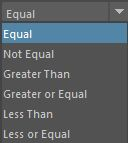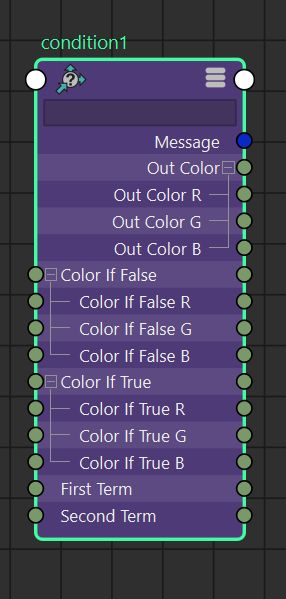BlendColors math node specification. Linear interpolation between Color1 and Color2 given the parameter $$blender \in [0.0, 1.0]$$.

 Output = Color1.rgb * (1.0 - blender) + Color2.rgb * blender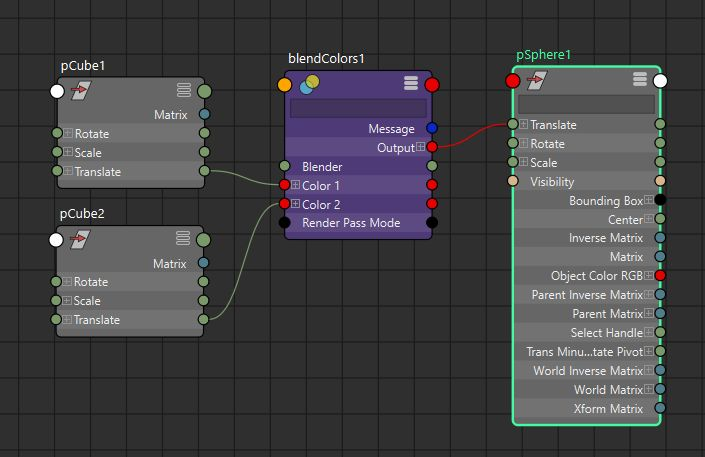Reverse math node specification. Reverse a parametric parameter: Output = 1.0 - Input.

 Output.x = 1.0 - Input.x     Output.y = 1.0 - Input.y     Output.z = 1.0 - Input.z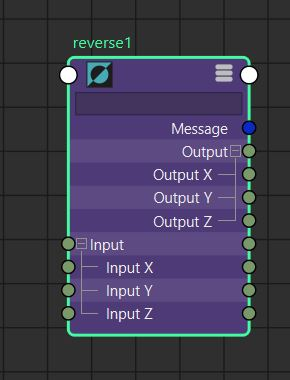Remaps an input scalar to an output scalar according to a user defined curve. Also outputs a color according to the position of the input scalar into the user defined color gradient.
Note: always prefer 'remapValue' which is really fast to evaluate contrary to the 'animCurve' node.
 OutputValue = Curve( InputValue ) Where the function "float Curve(float)" is the curve displayed in the attribute editor and which control points are stored in the "Value[]" attribute; OutputColor.RGB = Gradient( InputValue ) Where the function "Vec3 Gradient(float)" is the gradient strip displayed in the attribute editor and which interpolated colors are stored in the "Color[]" attribute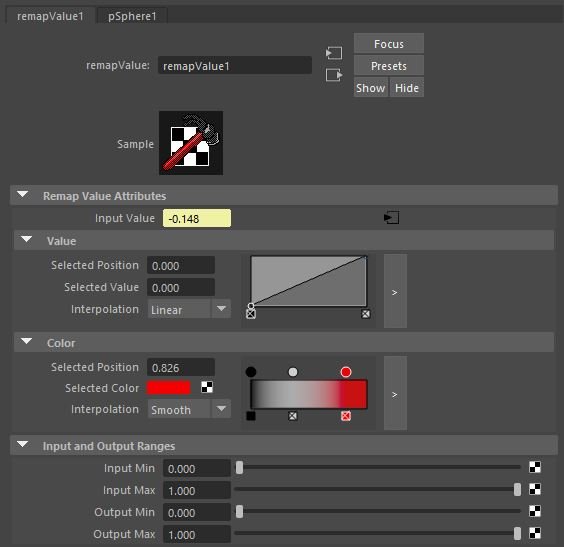Not covered here:
- pointMatrixMult   (matrix multiplication against vector or point)
- vectorProduct   (dot product, cross product, vector or point matrix product)
- clamp   (clamp(vec3, minVec3, maxVec3))
- multDoubleLinear (scalar multiplication: out = realValue1 * realValue2)
- pointConstraint can be used to compute the barycenter of several points (outPoint3 = ∑ point_i / n)

## Trigonometry

Although not natively present you can emulate trigonometry functions (sin, cos etc)

## Matrices

Official list of built in matrix nodes

Multiplication order, from right to left as we increase the index:

Indices:     n      ...        1       0
Matrix :  mat_n  *  ...  *  mat_1 * mat_0


Other nodes not covered here: addMatrix, aimMatrix, blendMatrix, composeMatrix, fourByFourMatrix, holdMatrix, passMatrix, pickMatrix, uvPin, proximityPin

Multiplies a list of 4x4 matrices together.
 $$MatrixSum = \prod_{i=n}^{0} MatrixIn[i]$$ MatrixSum = MatrixIn[n] * ... * MatrixIn * MatrixIn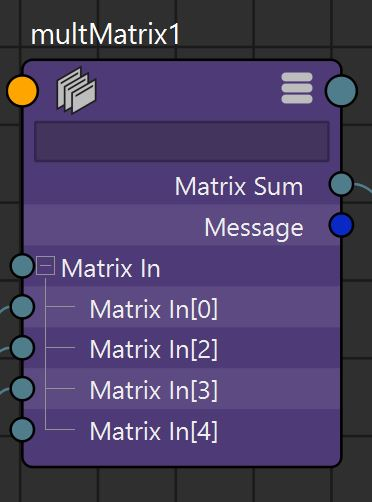Weighted sum of a list of 4x4 matrices.
 $$MatrixSum = \sum_{i=n}^{0} WtMatrix[i].MatrixIn * WtMatrix[i].WeightIn$$ MatrixSum = WtMatrix[n].MatrixIn * WtMatrix[n].WeightIn * ... * WtMatrix.MatrixIn * WtMatrix.WeightIn * WtMatrix.MatrixIn * WtMatrix.WeightIn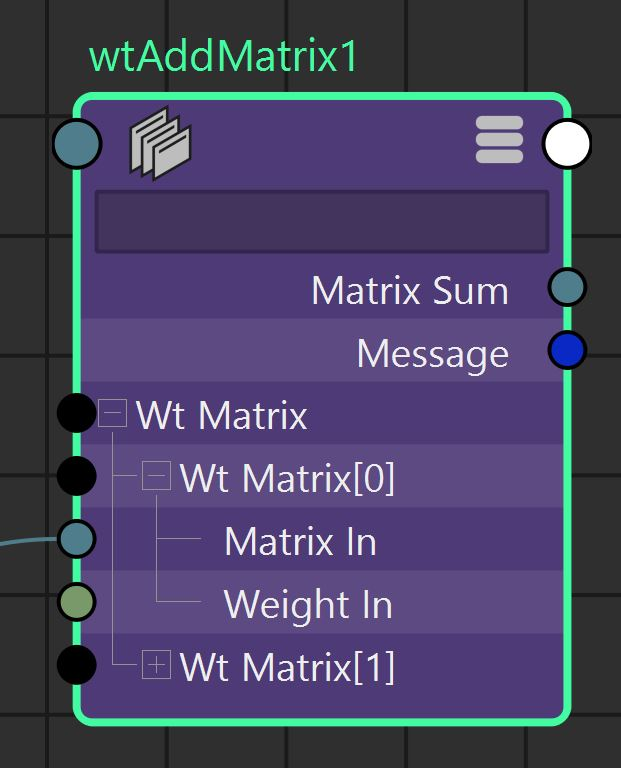Takes a 4x4 matrices and outputs its rotation, translation, scale, shear components.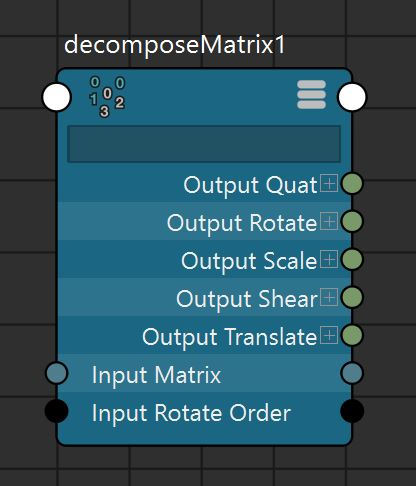Takes rotation, translation, scale, shear components and builds a 4x4 matrix.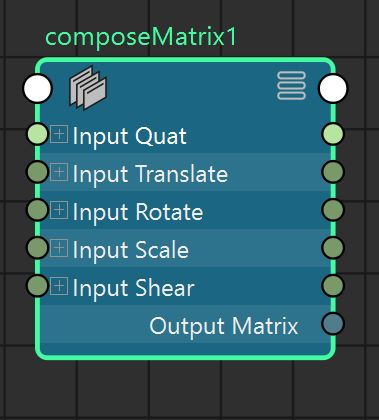Takes a 4x4 matrix in input and outputs a matrix with only certain components (e.g. translate, scale) of the input matrix. Which component is set to identity and which component's value will be picked is decided by the attributes useScale, useTranslate etc.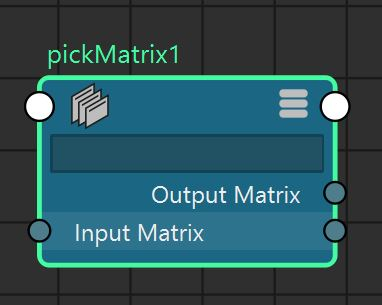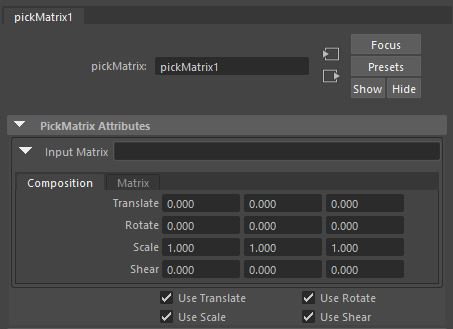Extra nodes in 'matrixNodes.mll' plugin:
- inverseMatrix node
- transposeMatrix node